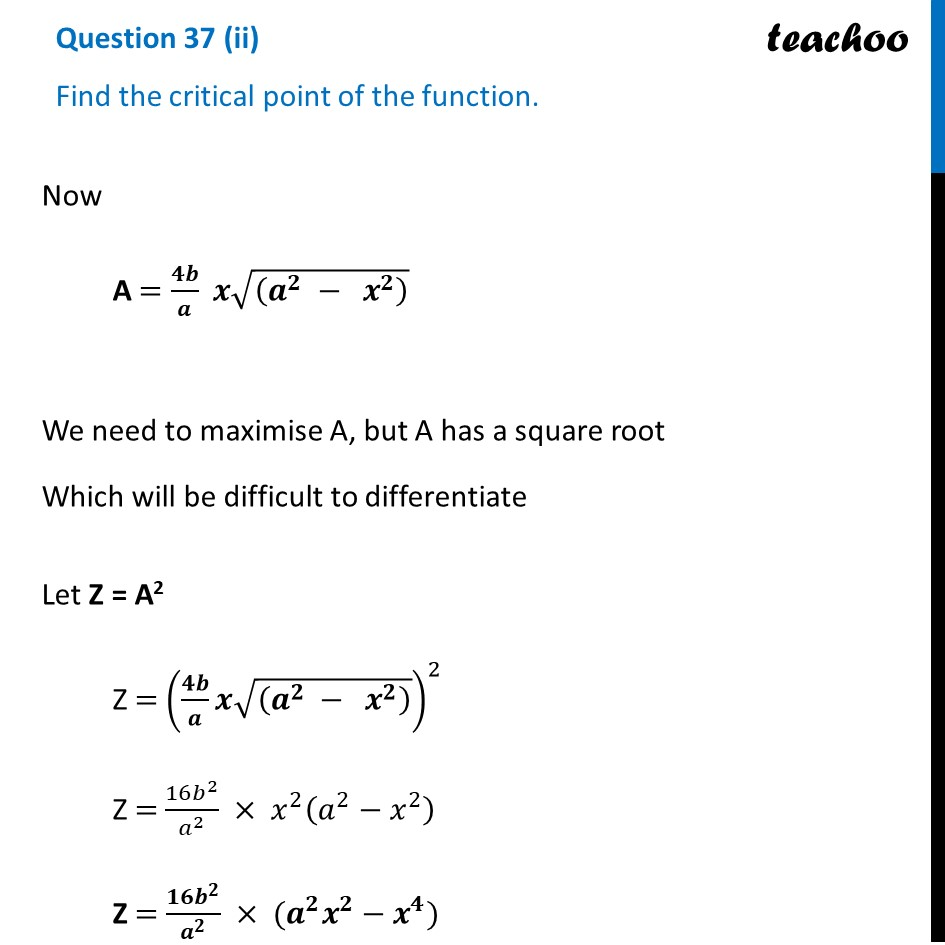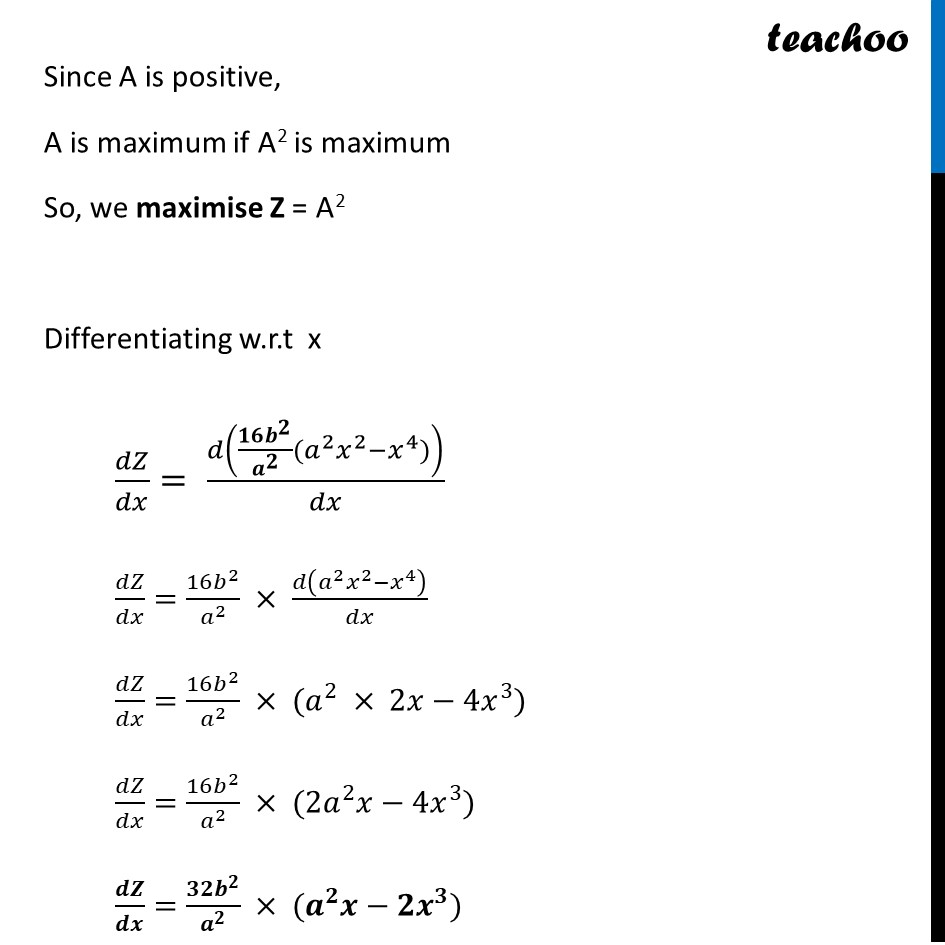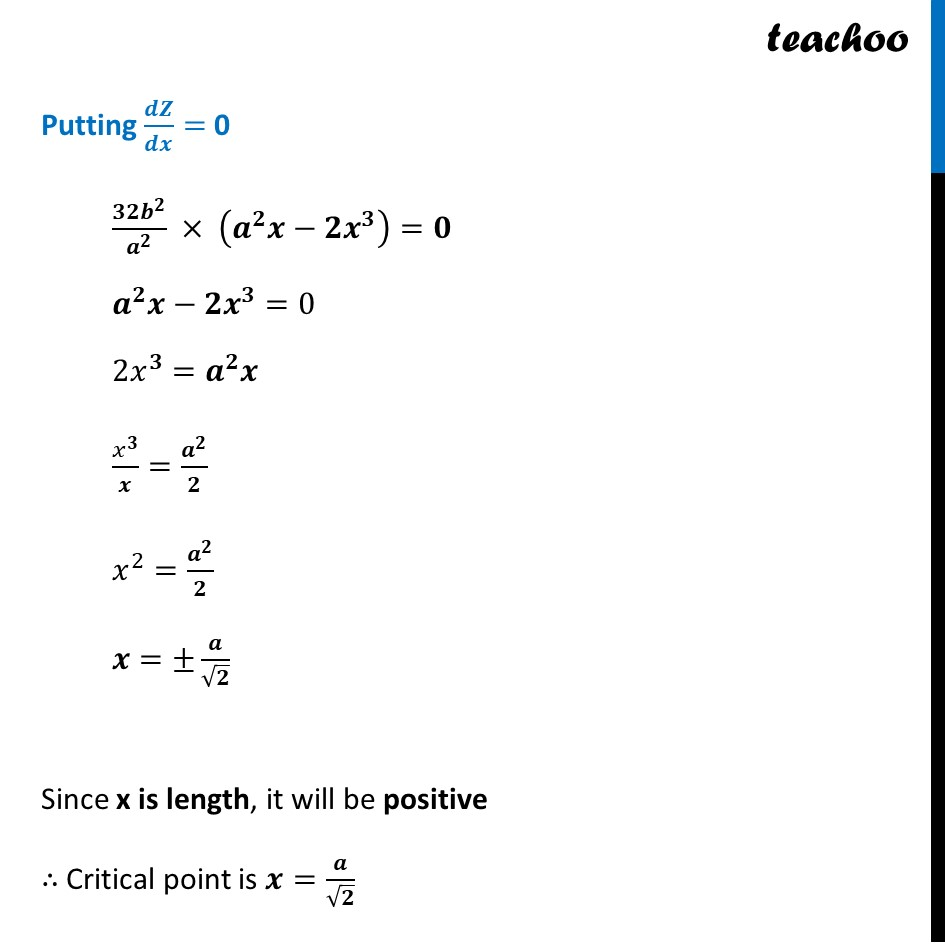CBSE Class 12 Sample Paper for 2023 Boards

Class 12
Solutions of Sample Papers and Past Year Papers - for Class 12 Boards

## Find the critical point of the function.Get live Maths 1-on-1 Classs - Class 6 to 12

### Transcript

Question 37 (ii) Find the critical point of the function.Now A =(" " 𝟒𝒃)/𝒂 " " 𝒙√((𝒂^𝟐 − 𝒙^𝟐 ) ) We need to maximise A, but A has a square root Which will be difficult to differentiate Let Z = A2 Z = ((" " 𝟒𝒃)/𝒂 𝒙√((𝒂^𝟐 − 𝒙^𝟐 ) ))^2 Z = (16𝑏^2)/𝑎^2 × 𝑥^2 (𝑎^2−𝑥^2 ) Z = (𝟏𝟔𝒃^𝟐)/𝒂^𝟐 × (𝒂^𝟐 𝒙^𝟐−𝒙^𝟒) Since A is positive, A is maximum if A2 is maximum So, we maximise Z = A2 Differentiating w.r.t x 𝑑𝑍/𝑑𝑥= 𝑑((𝟏𝟔𝒃^𝟐)/𝒂^𝟐 (𝑎^2 𝑥^2−𝑥^4))/𝑑𝑥 𝑑𝑍/𝑑𝑥=(16𝑏^2)/𝑎^2 × 𝑑(𝑎^2 𝑥^2−𝑥^4 )/𝑑𝑥 𝑑𝑍/𝑑𝑥=(16𝑏^2)/𝑎^2 × (𝑎^2 × 2𝑥−4𝑥^3) 𝑑𝑍/𝑑𝑥=(16𝑏^2)/𝑎^2 × (〖2𝑎〗^2 𝑥−4𝑥^3) 𝒅𝒁/𝒅𝒙=(𝟑𝟐𝒃^𝟐)/𝒂^𝟐 × (𝒂^𝟐 𝒙−𝟐𝒙^𝟑) Putting 𝒅𝒁/𝒅𝒙= 0 (𝟑𝟐𝒃^𝟐)/𝒂^𝟐 × (𝒂^𝟐 𝒙−𝟐𝒙^𝟑 )=𝟎 𝒂^𝟐 𝒙−𝟐𝒙^𝟑=0 2𝑥^𝟑=𝒂^𝟐 𝒙 𝑥^𝟑/𝒙=𝒂^𝟐/𝟐 𝑥^2=𝒂^𝟐/𝟐 𝒙=±𝒂/√𝟐 Since x is length, it will be positive ∴ Critical point is 𝒙=𝒂/√𝟐